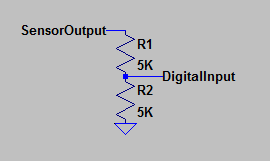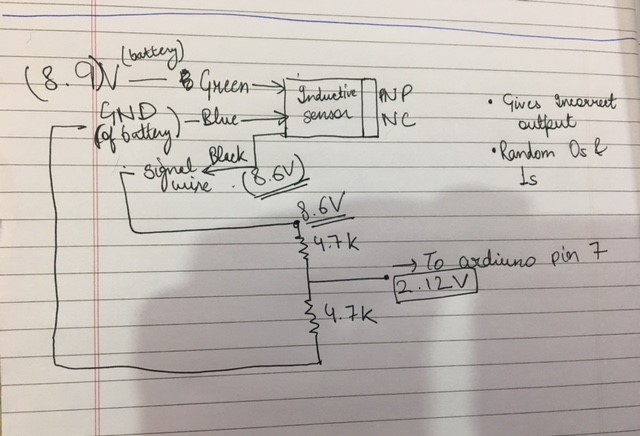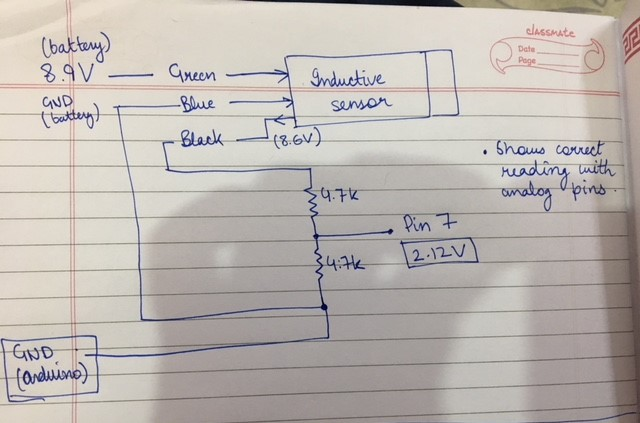# problems with inductive sensor

I brought LJ18A3-8-Z/BY Inductive sensor for a metal detecting robot. connections are:

black- digital input 7
brown- 9V
blue- gnd

when the sensor is brought close to a metal the led on the sensor lights up which indicates that the sensor is working but the signal sent to arduino is not correct.
I get a floating output of zeros and ones in arduino serial monitor.
the code i m using is

``````int val;
void setup()
{

Serial.begin(9600);
pinMode(7, INPUT);
pinMode(13, OUTPUT);
}

void loop()
{
delay(100);
Serial.println(val);
if(val == HIGH)
{
digitalWrite(13,HIGH);

}
else
{
digitalWrite(13,LOW);

}
}
``````

i have also checked the sensor output using a multimeter which shows:

8V when not metal is present and
0V when metal is present.

i have converted the 8v to 4v for input to the digital pin for safety of arduino. Still not working. please help ASAP.

i have converted the 8v to 4v for input to the digital pin for safety of arduino.

How? Post a wiring diagram (hand drawn, not a Fritzing mess).

i have attached the diagram.

[update] i also tried to run the ReadAnalogVoltage example by connecting to sesnor's output to A0 pin. doing this also showed bad values....not the one i m getting on multimeter.
i m not able to get the problem.

As you have discovered, that circuit won't work.

The sensor you have, according to this seller, has a PNP output, which must be connected to ground with a resistor.

To lower the output voltage to 0 to 5V for the Arduino, use a voltage divider as shown below.The sensor is intended to run on 10V, minimum.

Still the same output.
the sensor i received is a PNP NC because when no metal is detected the output is 12V when i gave it 12V supply.
when metal is present the output is 0V.

Sorry for the wrong information, i checked that on the sensor itself is LJ18A3-8-Z/BX.

the sensor i received is a PNP NC because when no metal is detected the output is 12V when i gave it 12V supply.
when metal is present the output is 0V.

In that case, the circuit I posted will work fine, except change the voltage divider to R1= 6.8K, R2= 4.7K to avoid >5V on the input pin.

The following statement contradicts the one above. But the above circuit will work in either case.

Sorry for the wrong information, i checked that on the sensor itself is LJ18A3-8-Z/BX.

I kind of found the problem. the problem is of ground. i have attached two images to show this.
All the voltage values are obtained from Multimeter.

First image is drawn with black ink(INCORRECT_CONNECTION_BLACK) which shows that:

the sensor output is not equal to sensor input i.e 8.9V.
the voltage divider should give output (8.9*0.5= 4.45) as R1=4.7k and R2=4.7k but shows output 2.12V

Second image is drawn with blue ink(CORRECT_BLUE):
When i connect GND of arduino to the GND of voltage divider the ReadAnalogVolage code it shows correct output i.e 2.12(but i want 4.5V o/p so it is read by digital pin).
Since i m unable to get correct output voltage from voltage divider i changed my code to

``````reading = analogRead(A4);
Serial.print("\n");
Serial.print("Metal in Proximity = ");
Serial.print(metal);
Serial.println(" %");
Serial.println("Metal Detected");
delay(100);
``````

this does the magic but i want the signal to be read on a digital pin.

i also directly connected the sensor output to pin 7 and is works there too for this code

the code i m using is

but for the safety of my arduino please help me to rectify my voltage divider output and it would be great if u could explain me why the arduino GND helped.

Forgive me if the images are shabby i m new to the forums.